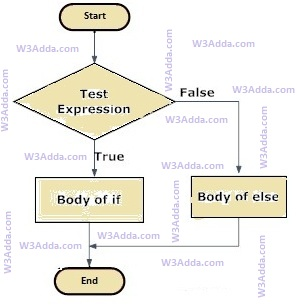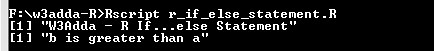# R if else Statement

In this tutorial you will learn about the R if else Statement and its application with practical example.

## R if else Statement

In R, when we want to execute a block of code when if condition is true and another block of code when if condition is false, In such a case we use if…else statement.

## R If…else Statement Flow DiagramR-if-else

Syntax:-

Here, Condition is a Boolean expression that results in either True or False, if it results in True then statements inside if body are executed, if it results in False then statements inside else body are executed.

Example:-

Output:-In this tutorial we have learn about the R if else Statement and its application with practical example. I hope you will like this tutorial.